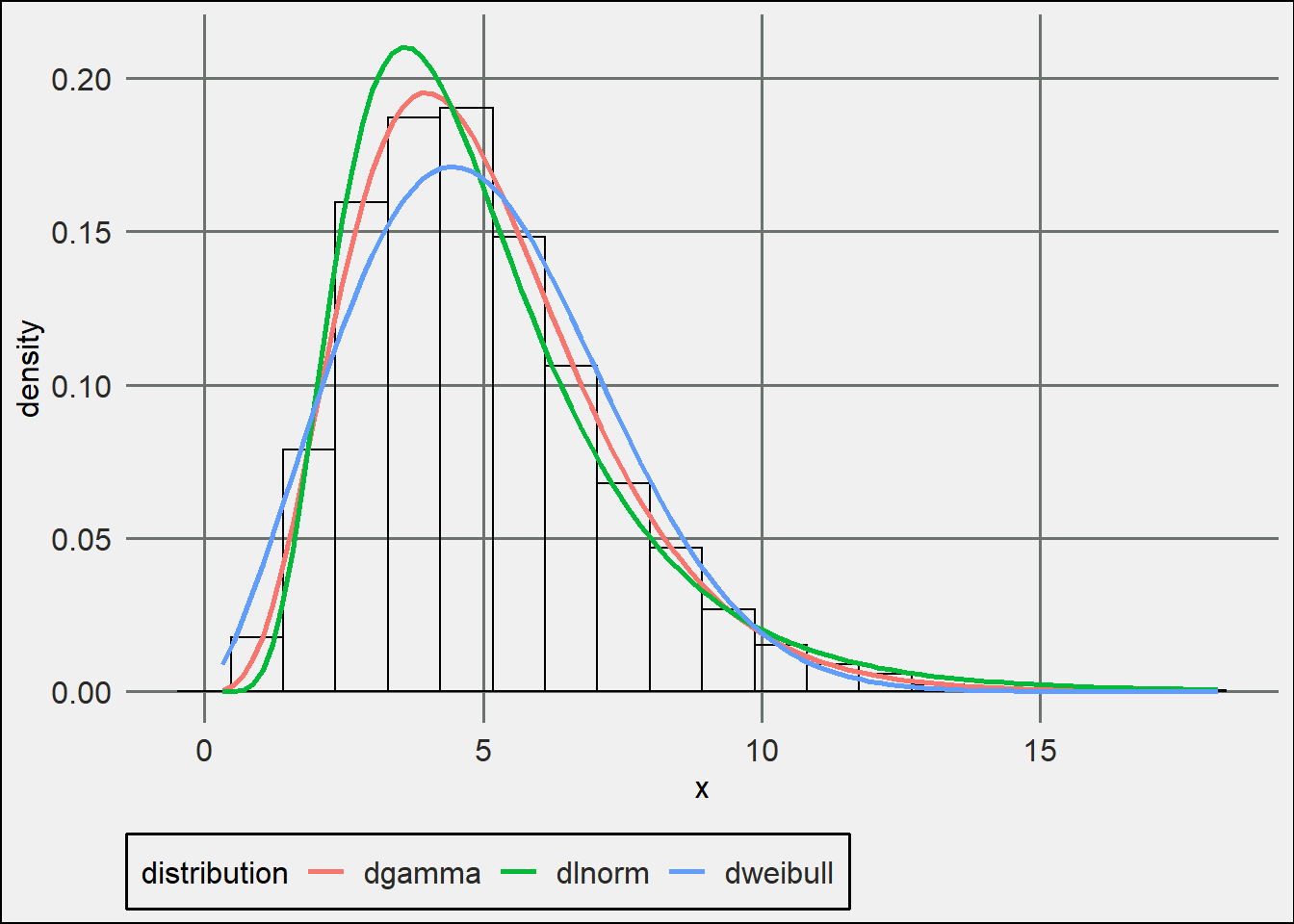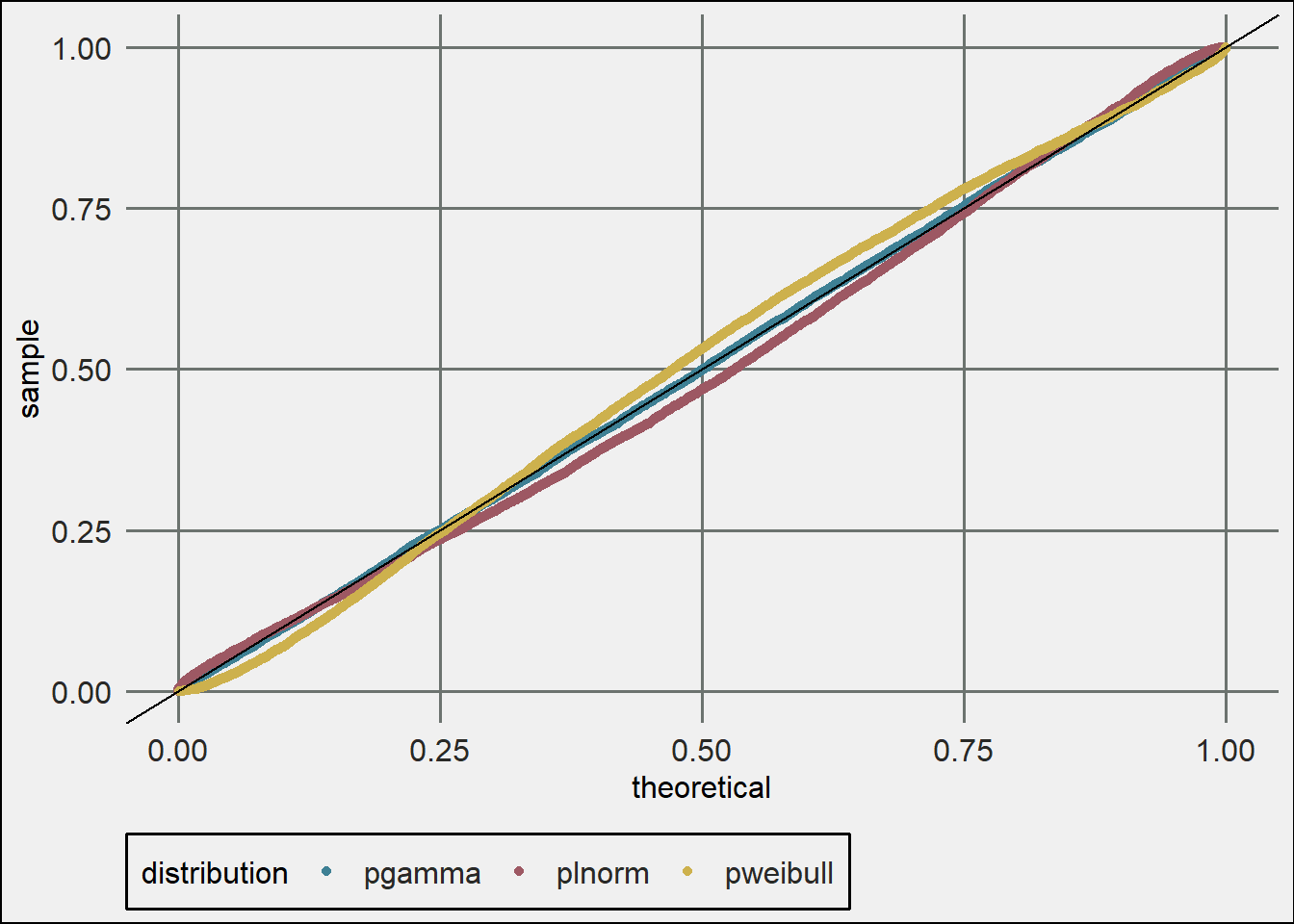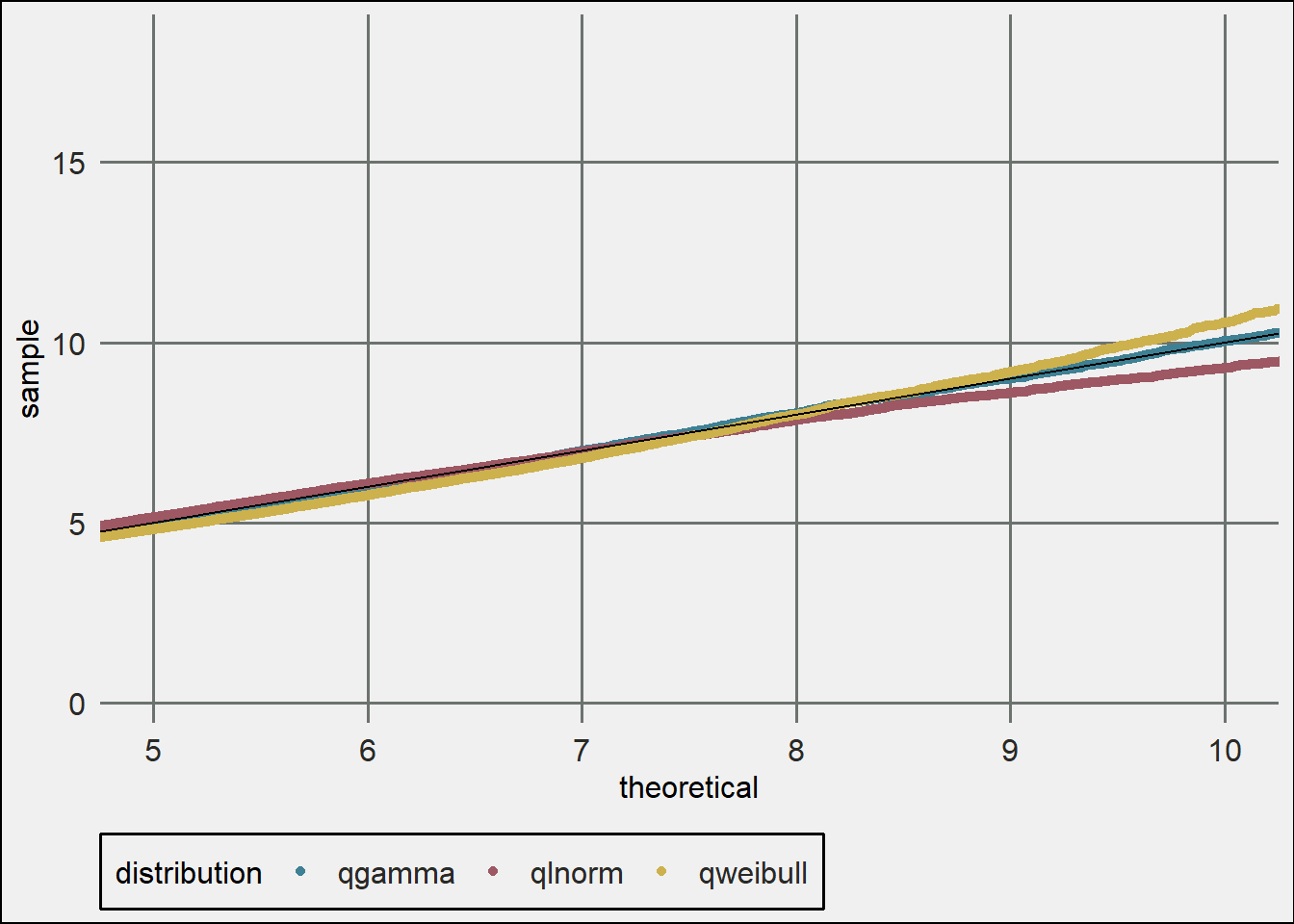• Fixed appearance of plots

• Added plot_density function for comparison pdfs of fitted functions

• Updated argument naming conventions

fitur on CRAN

## Density Diagnostic Plot

library(fitur)

## Warning: replacing previous import 'vctrs::data_frame' by 'tibble::data_frame'

library(ggplot2)
library(trstyles)
set.seed(37)
x <- rgamma(10000, 5)
dists <- c('gamma', 'lnorm', 'weibull')
fits <- lapply(dists, fit_univariate, x = x)
theme_set(theme_tr())
plot_density(x, fits, nbins = 20)## QQ-Plot

The output is a ggplot object so you can add colors, styling, etc.

plot_pp(x, fits) +
scale_color_tr()## PP-Plot

You can also zoom in on different areas.

plot_qq(x, fits) +
scale_color_tr() +
coord_cartesian(xlim = c(5,10))